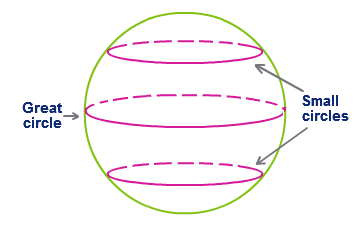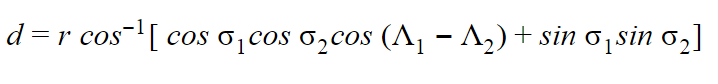# Great Circle Formula

The largest circle that can be drawn on the sphere surface is the great circle. The shortest distance between any two points on the sphere surface is the Great Circle distance. Historically, the Great circle is also called as an Orthodrome or Romanian Circle.

The diameter of any sphere coincides with the diameter of the great circle. The great circle is used in the navigation of ship or aircraft. The idea that is the Earth is somewhat spherical helps in navigating as we come to know for the shortest distance in the sphere. Pretty much useful, isn’t it?Great circle formula is given by,Where,
r is the radius of the earth

σ  is the latitude
∆ is the longitude

### Solved Example

Question: Find the great circle distance if the radius is 4.7 km, the latitude is (45o, 32o) and longitude is (24o, 17o).

Solution:

Given,

$$\begin{array}{l}\sigma_{1},\sigma_{2}=45^{\circ},32^{\circ}\end{array}$$
$$\begin{array}{l}\Lambda_{1},\Lambda_{2}=24^{\circ},17^{\circ}\end{array}$$

r=4.7

Using the above given formula,

$$\begin{array}{l}d=4700\;cos^{-1}(0.52\times 0.83\times 0.75)+(0.85 \times 0.32)\end{array}$$

$$\begin{array}{l}d=4700\times 0.99\end{array}$$

D = 4653 m